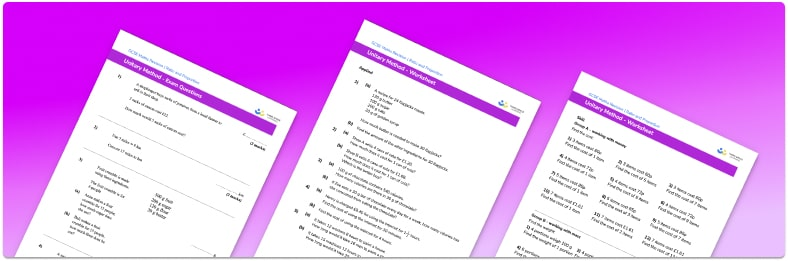# Unitary Method Worksheet• Section 1 of the unitary method worksheet contains 24 skills-based unitary method questions, in 3 groups to support differentiation
• Section 2 contains 5 applied unitary method questions with a mix of word problems and deeper problem solving questions
• Section 3 contains 4 foundation and higher level GCSE exam style unitary method questions
• An answer key and a mark scheme for all unitary method questions are provided
• Questions follow variation theory with plenty of opportunities for students to work independently at their own level
• All questions created by fully qualified expert secondary maths teachers
• Suitable for GCSE maths revision for AQA, OCR and Edexcel exam boards

• This field is for validation purposes and should be left unchanged.

You can unsubscribe at any time (each email we send will contain an easy way to unsubscribe). To find out more about how we use your data, see our privacy policy.

### Unitary method at a glance

The unitary method is a method that involves finding the value of a single unit and using that to find the value of a different number of units of something. For example, if a factory produces 3000 tubes of toothpaste in 4 days, we can find the number of tubes of toothpaste produced in 5 days using the unitary method. First we calculate the number of tubes of toothpaste in 1 day, 3000 divided by 4 = 750. We can then multiply by 5 to find the number produced in 5 days: 750 times 5 = 3750.

The above example has two variants with direct variation, commonly referred to as direct proportional. The unitary method can also be used for problems involving indirect variation (inverse proportion). For example, if a project is 6 days work for 3 people, we can work out how many days work it would be for 2 people. First, we find the number of days for 1 person, 6 times 3 = 18.  We can then divide by 2 to find the number of days for 2 people: 18 divided by 2 = 9.

Looking forward, students can then progress to additional ratio and proportion worksheets, for example aor aFor more teaching and learning support on Ratio and Proportion our GCSE maths lessons provide step by step support for all GCSE maths concepts.

## Do you have KS4 students who need more focused attention to succeed at GCSE?There will be students in your class who require individual attention to help them succeed in their maths GCSEs. In a class of 30, it’s not always easy to provide.

Help your students feel confident with exam-style questions and the strategies they’ll need to answer them correctly with our dedicated GCSE maths revision programme.

Lessons are selected to provide support where each student needs it most, and specially-trained GCSE maths tutors adapt the pitch and pace of each lesson. This ensures a personalised revision programme that raises grades and boosts confidence.

Find out more